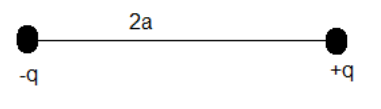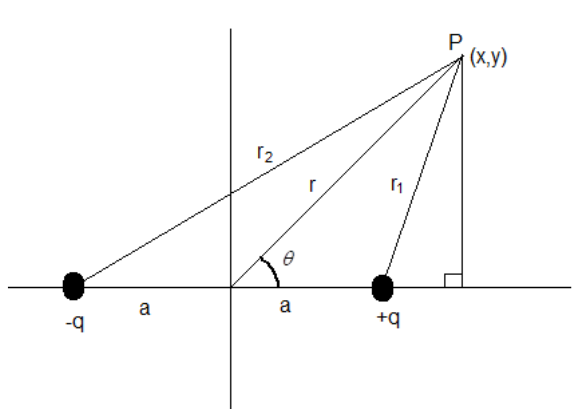Courses
Courses for Kids
Free study material
Free LIVE classes
More# Derive an expression for electric potential at point due to an electric dipole.Discuss the special cases.

Last updated date: 29th Mar 2023
Total views: 240.9k
Views today: 4.32kVerified
240.9k+ views
Hint: An electric dipole is a pair of two charges equal in magnitude (q) but opposite in nature (i.e. one is a positive charge and other is a negative charge). The two charges are separated by a distance of length 2a.

Formula used: $V=\dfrac{Kq}{r}$
${{(1\pm x)}^{n}}\approx 1\pm nx$, if x is very much small.

An electric dipole is a pair of two charges equal in magnitude (q) but opposite in nature (i.e. one is a positive charge and other is a negative charge). The two charges are separated by a distance of length 2a.
An electric dipole has a quantity called its dipole moment given by P=2qa.
A sketch of an electric dipole is shown below.Let an electric dipole with charges +q and –q lie on x-axis with the origin as the midpoint of the dipole.
Consider a point P at the coordinates (x,y). The distance of the point from origin be r making an angle $\theta$ with positive x-axis.Electric potential due to a charge q at a point, which is at a distance r from the charge is given as $V=\dfrac{Kq}{r}$ , Where V is the potential due to the charge and K is permittivity of free space. Now, the electric potential at point P will be due two charges (+q and -q).
Let the potential due to charge +q be ${{V}_{1}}$ .
Let the potential due to charge -q be ${{V}_{2}}$ .
Let the total electric potential due to both the charges be V.
Since, electric potential is a scalar quantity, $V={{V}_{1}}+{{V}_{2}}$.
If you see the given figure,
${{V}_{1}}=\dfrac{Kq}{{{r}_{1}}}$ ………………(i).
Since, is right-angled triangle, ${{r}_{1}}^{2}={{(x-a)}^{2}}+{{y}^{2}}$.
$\Rightarrow {{r}_{1}}=\sqrt{{{(x-a)}^{2}}+{{y}^{2}}}$
Substitute the value of ${{r}_{1}}$ in equation (i).
Therefore, ${{V}_{1}}=\dfrac{Kq}{\sqrt{{{(x-a)}^{2}}+{{y}^{2}}}}$.
And
${{V}_{2}}=-\dfrac{Kq}{{{r}_{2}}}$ ………. (ii).
Is also a right-angled triangle. Therefore, ${{r}_{2}}^{2}={{(x+a)}^{2}}+{{y}^{2}}$.
$\Rightarrow {{r}_{2}}=\sqrt{{{(x+a)}^{2}}+{{y}^{2}}}$
Substitute the value of ${{r}_{2}}$ in equation (ii).
Therefore, ${{V}_{2}}=\dfrac{-Kq}{\sqrt{{{(x+a)}^{2}}+{{y}^{2}}}}$.
This implies that $V={{V}_{1}}+{{V}_{2}}=\dfrac{Kq}{\sqrt{{{(x-a)}^{2}}+{{y}^{2}}}}-\dfrac{Kq}{\sqrt{{{(x+a)}^{2}}+{{y}^{2}}}}$
$V=Kq\left( \dfrac{1}{\sqrt{{{(x-a)}^{2}}+{{y}^{2}}}}-\dfrac{1}{\sqrt{{{(x+a)}^{2}}+{{y}^{2}}}} \right)$ …….(iii).
Consider the expression $\sqrt{{{(x\pm a)}^{2}}+{{y}^{2}}}$.
Open up the brackets.
$\Rightarrow \sqrt{{{x}^{2}}\pm 2ax+{{a}^{2}}+{{y}^{2}}}$. …………... (1)
But ${{x}^{2}}+{{y}^{2}}={{r}^{2}}$.
Therefore, expression (1) can be written as $\sqrt{{{r}^{2}}\pm 2ax+{{a}^{2}}}$.
Since r>>>>a, $\sqrt{{{r}^{2}}\pm 2ax+{{a}^{2}}}\approx \sqrt{{{r}^{2}}\pm 2ax}$.
From the figure we know, $x=rcos\theta$. Substitute the value of x in the above equation.
Therefore, $\sqrt{{{r}^{2}}\pm 2ax}=\sqrt{{{r}^{2}}\pm 2ar\cos \theta }$.
Take ${{r}^{2}}$ as a common term.
$\Rightarrow \sqrt{{{r}^{2}}\pm 2ar\cos \theta }=r\sqrt{1\pm \dfrac{2a\cos \theta }{r}}$ …….(2).
Here, $\dfrac{2a\cos \theta }{r}$ is a very much small value because r>>>>a.
Any term ${{(1\pm x)}^{n}}\approx 1\pm nx$, if x is a very much small value or almost equal to zero.
Let us use the same concept in equation (2).
Therefore, $r\sqrt{1\pm \dfrac{2a\cos \theta }{r}}=r{{\left( 1\pm \dfrac{2a\cos \theta }{r} \right)}^{\dfrac{1}{2}}}\approx r\left( 1\pm \dfrac{1}{2}\left( \dfrac{2a\cos \theta }{r} \right) \right)=r\left( 1\pm \dfrac{a\cos \theta }{r} \right)=r\pm a\cos \theta$.
Hence, we get that $\sqrt{{{(x\pm a)}^{2}}+{{y}^{2}}}\approx r\pm a\cos \theta$
Therefore, $\sqrt{{{(x+a)}^{2}}+{{y}^{2}}}\approx r+a\cos \theta$ and $\sqrt{{{(x-a)}^{2}}+{{y}^{2}}}\approx r-a\cos \theta$
Thence we can write equation (iii) as
$V=Kq\left( \dfrac{1}{r-a\cos \theta }-\dfrac{1}{r+a\cos \theta } \right)$
$\Rightarrow V=Kq\left( \dfrac{r+a\cos \theta -\left( r-a\cos \theta \right)}{\left( r-a\cos \theta \right)\left( r+a\cos \theta \right)} \right)$
$\Rightarrow V=Kq\left( \dfrac{r+a\cos \theta -r+a\cos \theta }{\left( {{r}^{2}}-{{a}^{2}}{{\cos }^{2}}\theta \right)} \right)$
$\Rightarrow V=Kq\left( \dfrac{2a\cos \theta }{\left( {{r}^{2}}-{{a}^{2}}{{\cos }^{2}}\theta \right)} \right)$.
We know that 2qa=P.
$\Rightarrow V=\dfrac{KP\cos \theta }{{{r}^{2}}-{{a}^{2}}{{\cos }^{2}}\theta }$ ……..(iv).
Therefore, the electric potential due to an electric dipole at a given point is equal to $\dfrac{KP\cos \theta }{{{r}^{2}}-{{a}^{2}}{{\cos }^{2}}\theta }$.
Special cases:
(i) When the given point is on the axial line of the dipole (i.e. $\theta =0$).
Substitute $\theta =0$ in equation (iv).
Therefore, $V=\dfrac{KP\cos 0}{{{r}^{2}}-{{a}^{2}}{{\cos }^{2}}0}$.
We know $\cos 0=1$.
Hence, $V=\dfrac{KP\cos 0}{{{r}^{2}}-{{a}^{2}}{{\cos }^{2}}0}=\dfrac{KP}{{{r}^{2}}-{{a}^{2}}}$
(ii) When the given point is on the equatorial axis of the dipole (i.e. $\theta =\dfrac{\pi }{2}$)
Substitute $\theta =\dfrac{\pi }{2}$ in equation (iv).
Therefore, $V=\dfrac{KP\cos \left( \dfrac{\pi }{2} \right)}{{{r}^{2}}-{{a}^{2}}{{\cos }^{2}}\left( \dfrac{\pi }{2} \right)}$.
We know $\cos \left( \dfrac{\pi }{2} \right)=0$.
Hence, $V=\dfrac{KP\cos \left( \dfrac{\pi }{2} \right)}{{{r}^{2}}-{{a}^{2}}{{\cos }^{2}}\left( \dfrac{\pi }{2} \right)}=\dfrac{KP(0)}{{{r}^{2}}-{{a}^{2}}(0)}=0$.
Therefore, when the given point is on the equatorial axis, the electric potential is zero.

Note: You may be thinking about the condition on r that it should be very much greater than a. there is a valid reason for this condition. We study the concept of dipole because molecules exist in the form of dipoles. For example, the bond between oxygen atom and hydrogen atom in water molecules is a dipole moment. You may know that the distance between these atoms is very small and therefore it is negligible when compared to the distance r, from its midpoint.